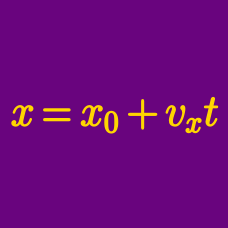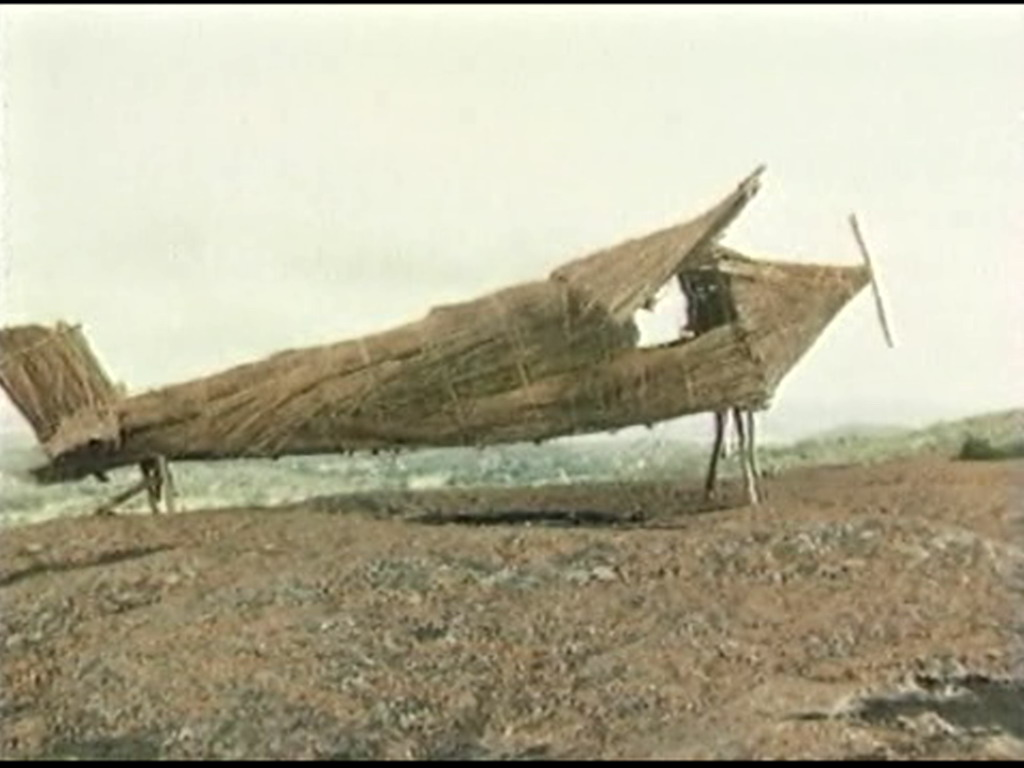Classical Mechanics

# 2D Kinematics - Problem SolvingAn airplane is taking off on the runway. At the moment the wheels leave the ground, the plane is traveling at $60 \text{ m/s}$ horizontally. The wings generate a lift which causes a vertical acceleration of $1 \text{ m/s}^2 ,$ and the jets produce a horizontal acceleration of $2 \text{ m/s}^2 .$ When the airplane is flying at an altitude of $3200 \text{ m},$ what is its horizontal distance from the airport?

The air resistance is negligible.

A hunter aims directly at a target $66 \text{ m}$ away, at the same height as the gun. If the bullet is fired at a speed of $66 \text{ m/s},$ by how much will the bullet miss the target?

NOTES: The air resistance is negligible, and the gravitational acceleration is $g=10\text{ m/s}^2.$ You may also assume the bullet does not hit the ground until after passing the target.

A boy throws a ball at $32 \sqrt{2} \text{ m/s}$ at a $45^ \circ$ angle with the ground. Find the horizontal distance the ball flies.

Gravitational acceleration is $g =10 \text{ m/s}^2,$ and air resistance is negligible.

A ball is thrown horizontally at $16 \text{ m/s} .$ If the ball travels a horizontal distance of $80 \text{ m}$ before hitting the ground, from what height was the ball thrown?

The gravitational acceleration is $g= 10 \text{ m/s}^2.$

A car initially at rest accelerates uniformly at a rate of $(6 \text{ m/s}^2) \hat{i}.$ If the car travels $(108 \text{ m}) \hat{i} ,$ what is its final velocity?

×